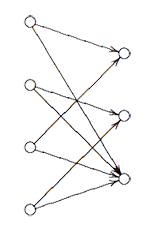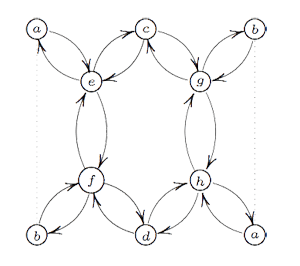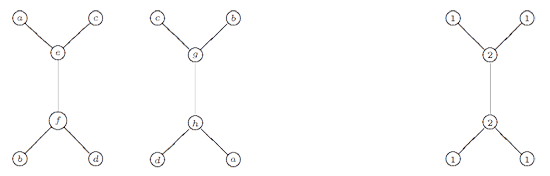Hexagons keep on popping up in the representation theory of the modular group and its close associates. We have seen before that singularities in 2-dimensional representation varieties of the three string braid group $B_3$ are ‘clanned together’ in hexagons and last time Ive mentioned (in passing) that the representation theory of the modular group is controlled by the double quiver of the extended Dynkin diagram $\tilde{A_5}$, which is an hexagon…

Today we’re off to find representations of the extended modular group $\tilde{\Gamma} = PGL_2(\mathbb{Z})$, which is obtained by adding to the modular group (see this post for a proof of generation)

$\Gamma = \langle U=\begin{bmatrix} 0 & -1 \\\ 1 & 0 \end{bmatrix},V=\begin{bmatrix} 0 & 1 \\\ -1 & 1 \end{bmatrix} \rangle$ the matrix $R=\begin{bmatrix} 0 & 1 \\\ 1 & 0 \end{bmatrix}$

In terms of generators and relations, one easily verfifies that

$\tilde{\Gamma} = \langle~U,V,R~|~U^2=R^2=V^3=(RU)^2=(RV)^2=1~\rangle$

and therefore $\tilde{\Gamma}$ is the amalgamated free product of the dihedral groups $D_2$ and $D_3$ over their common subgroup $C_2 = \langle~R~\rangle$, that is

$\tilde{\Gamma} = \langle U,R | U^2=R^2=(RU)^2=1 \rangle \ast_{\langle R | R^2=1 \rangle} \langle V,R | V^3=R^2=(RV)^2=1 \rangle = D_2 \ast_{C_2} D_3$

From this description it is easy to find all n-dimensional $\tilde{\Gamma}$-representations $V$ and relate them to quiver-representations. $D_2 = C_2 \times C_2$ and hence has 4 1-dimensonal simples $S_1,S_2,S_3,S_4$. Restricting $V\downarrow_{D_2}$ to the subgroup $D_2$ it decomposes as

$V \downarrow_{D_2} \simeq S_1^{\oplus a_1} \oplus S_2^{\oplus a_2} \oplus S_3^{\oplus a_3} \oplus S_4^{\oplus a_4}$ with $a_1+a_2+a_3+a_4=n$

Similarly, because $D_3=S_3$ has two one-dimensional representations $T,S$ (the trivial and the sign representation) and one simple 2-dimensional representation $W$, restricting $V$ to this subgroup gives a decomposition

$V \downarrow_{D_3} \simeq T^{b_1} \oplus S^{\oplus b_2} \oplus W^{\oplus b_3}$, this time with $b_1+b_2+2b_3=n$

Restricting both decompositions further down to the common subgroup $C_2$ one obtains a $C_2$-isomorphism $V \downarrow_{D_2} \rightarrow^{\phi} V \downarrow_{D_3}$ which implies also that the above numbers must be chosen such that $a_1+a_3=b_1+b_3$ and $a_2+a_4=b_2+b_3$. We can summarize all this info about $V$ in a representation of the quiverHere, the vertex spaces on the left are the iso-typical factors of $V \downarrow_{D_2}$ and those on the right those of $V \downarrow_{D_3}$ and the arrows give the block-components of the $C_2$-isomorphism $\phi$. The nice things is that one can also reverse this process to get all $\tilde{\Gamma}$-representations from $\theta$-semistable representations of this quiver (having the additional condition that the square matrix made of the arrows is invertible) and isomorphisms of group-representation correspond to those of quiver-representations!

This proves that for all n the varieties of n-dimensional representations $\mathbf{rep}_n~\tilde{\Gamma}$ are smooth (but have several components corresponding to the different dimension vectors $~(a_1,a_2,a_3,a_4;b_1,b_2,b_3)$ such that $\sum a_i = n = b_1+b_2+2b_3$.

The basic principle of _M-geometry_ is that a lot of the representation theory follows from the ‘clan’ (see this post) determined by the simples of smallest dimensions. In the case of the extended modular group $\tilde{\Gamma}$ it follows that there are exactly 4 one-dimensional simples and exactly 4 2-dimensional simples, corresponding to the dimension vectors

$\begin{cases} a=(0,0,0,1;0,1,0) \\\ b=(0,1,0,0;0,1,0) \\\ c=(1,0,0,0;1,0,0) \\\ d=(0,0,1,0;1,0,0) \end{cases}$ resp. $\begin{cases} e=(0,1,1,0;0,0,1) \\\ f=(1,0,0,1;0,0,1) \\\ g=(0,0,1,1;0,0,1) \\\ h=(1,1,0,0;0,0,1) \end{cases}$If one calculates the ‘clan’ of these 8 simples one obtains the double quiver of the graph on the left. Note that a and b appear twice, so one should glue the left and right hand sides together as a Moebius-strip. That is, the clan determining the representation theory of the extended modular group is a Moebius strip made of two hexagons!

However, one should not focuss too much on the hexagons (that is, the extended Dynkin diagram $\tilde{A_5}$) here. The two ‘backbones’ (e–f and g–h) have their vertices corresponding to 2-dimensional simples whereas the topand bottom vertices correspond to one-dimensional simples. Hence, the correct way to look at this clan is as two copies of the double quiver of the extended Dynkin diagram $\tilde{D_5}$ glued over their leaf vertices to form a Moebius strip. Remark that the components of the sotropic root of $\tilde{D_5}$ give the dimensions of the corresponding $\tilde{\Gamma}$ simples.The remarkable ubiquity of (extended) Dynkins never ceases to amaze!

## One Comment

This site uses Akismet to reduce spam. Learn how your comment data is processed.